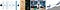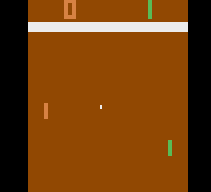# How AI Is Beating Humans at Games

## Understanding the basics of Reinforcement Learning and Deep Q Networks (DQN) for AI

You may have heard of other applications of AI, such as pattern recognition (detecting cancers, etc.). All of these applications rely on supervised learning where the computer is given a labeled dataset (it knows that is right and wrong). These applications are great but face a few shortcomings. Since they are trained off of datasets, they will simply recognize patterns and exploit those rather than exploring where humans have not.Some of the places where reinforcement learning is being applied today. Currently, it is mainly deployed in smart robotics and games. Credit: Andrej Karpathy

This is where reinforcement learning comes in. It is neither supervised or unsupervised learning; it is its subset of AI. Reinforcement learning relies on a concept that is very intuitive to humans — trial and error. Positive actions and behaviours are reinforced by providing rewards. Mostly, all the computer is trying to do is maximize the prizes in any given circumstance

This approach to AI is not considered under supervised or unsupervised learning and is instead its paradigm. Currently, deep learning (using neural networks) has been getting all the attention; however, it requires a labelled dataset. This can be problematic as a high-quality dataset that is large enough is hard to come by for specific AI applications. This makes it ideal for RL as it does not require any past information regarding its environment.

One of the reasons I find RL exciting because of its development in Artificial General Intelligence. Currently, most applications of AI refer to narrow AI. This means that AI excels at only doing a particular task. While this may seem great, its a far cry from achieving AGI like the ones seen in Sci-Fi movies. However, due to the nature of RL, it is claimed as one of the best approaches to achieving AGI as it mimics the general learning approach of humans.

Let’s say you were playing a brand new video that looked like Packman. When loading up the game, you got particularly impatient and just skipped through the screen, which showed the rules and object of the game. You have just loaded in the first round of the game, but you don’t know what to do.

Except, there is a score at the top, and you are trying to get the highest score possible. You have no clue how. So you start moving around. You suddenly get hit by a ghost, and you lose points. Now you know that you probably don’t want to go anywhere near a ghost. Then you come across a couple of coins, and you see your score rapidly increase. Now you know that you need to get as many coins as you can without being caught by a ghost. So you start doing that. You are able to the maximum amount of coins. However, you notice a door like contraption on the other side of the game area. So, in addition to exploiting all the coins, you should also explore that door.

# Components of Reinforcement Learning

The system that I mentioned above is mimicking that of reinforcement learning. All reinforcement learning systems are made up of 4 essential elements:

• An Agent (You): is placed in an environment and tries to maximize the reward
• An Environment (the game level): These act as the boundaries as doe things the agent can and cannot do.
• A Policy (brain) it defines the agent’s behaviour in a particular state by examining the various probabilities
• A Rewards Function: it sets the goal of the learning that is taking place. It awards a numerical reward. As mentioned before, the purpose of the agent is to maximize the reward immediately.
• A Value Function: While a reward function is excellent for guiding short term actions, the value function helps optimize for the long run. It tries to figure out what behaviour yields the highest rewards in the long run.
• A Q-value function: it can be thought of as a combination of the value and reward function. Based on the chosen action, it determines what state has the best Q value (long term reward).
• A Model: diff between model and environment?

# Everything is a Math problem!

While it may be easy just to conceptualize the reward and states experienced by the agent, it is crucial to recognize that it is a complicated math problem. The whole process of reinforcement learning is known collectively as Markov Processes, which can be broken down into Markov Reward Processes, Bellman Equation, and Markov Decision Processes.

Warning: Knowing exactly how RL works is not necessary to follow along for the rest of the article or project, but builds a strong base of knowledge to venture deeper into RL.

## The Markov Property

The Markov Property states that:

“ The future is independent of the past given the present.”

This is important because once the agent knows the current state of the environment, it can discard all information about the past (much more memory efficient). Any state that has the Markov property is considered a Markov. From there, a transition function represents the probability distribution of future states given the present state.

## The Markov Process

The Markov process simply refers to a process that does not rely on prior states, but instead states with the Markov property. A Markov process is defined as (S, P) where S is the state space, and P is the transition function of the Markov state.

## Markov Reward Process (MRP)

It is a Markov process that determines the amount of reward accumulated based upon being a sequence of another Markov process. An MRP is defined similar to a Markov Process but with more parameters:

(S, P, R, 𝛾). In addition to S being the state space and P being the transition function, R is the reward function, which defines the amount of immediate reward the agent will get and 𝛾 being the discount factor. The discount factor tells the agent how to value immediate and long term rewards. For example, a value of 0 makes the agent optimize solely for short term rewards whereas a factor of 1 makes the agent put maximum emphasis on long-term rewards

## Bellman Equation

To maximize the sum of the rewards given in a particular state, the agent must know the optimal value of the function to get the maximum sum of all possible rewards. To do so, we can use the Bellman equation to calculate the value function for each given state. It is broke down further into an immediate value reward (ignores discount factor) and the discounted value function (includes the discount factor)

Directly implementing the Bellman equation is very memory inefficient as the complexity of the calculation increases linearly. This is fine for most small MRPs; however, other methods like Dynamic Programming, Monte-Carlo and Temporal Difference learning are used for scalable and efficient solutions.

## Markov Decision Process (MDP)

The Markov Decision Process (MDP) can be seen as an MRP involving decisions and in an environment consisting of Markov states. The Bellman equation is implemented here as the point of RL is to maximize the MDP. An MDP is defined as a function with inputs (S, A, P, R, 𝛾), where A is defined as the limited options of actions (S, P, R, and 𝛾 are described in the MRP). Primarily, the MDP produces a value function that outputs the predicted reward of any action from the set.

## And It Doesn’t Stop There

While we have covered the very basics of the MDP, there are many other processes within MPs such as Bellman optimality equation, optimal state-value function, optimal action-value function and overall optimal policy.

Check out Richard Sutton’s Introduction to Reinforcement Learning to go even more in-depth into Markov Processes

# Q-Learning

Q-Learning is a popular RL algorithm used for a variety of problems. It is different than other algorithms because it is an off-policy algorithm. This means that it is separate from the primary policy and only receives the action from the central policy.

Once it receives an action, it tries to optimize for the Q-value. This is called a greedy algorithm because it is only trying to maximize one thing, the Q-value.

# Deep Q Learning

Now actually implementing Q-learning algorithms has proven to have its downsides. The biggest downside is the lack of generalization. In other words, the agent has no clue what to do in an unseen state, even if it is very similar to known states.

## What’s the solution?

Neural networks. A deep neural network is used to implement the algorithm to increase the agent’s ability to generalize. The architecture of the Neural Network is relatively straightforward. In this case, you can feed the game frames into Convolution layers for edge recognition. Then, a series of fully connected layers can take that information and output Q-values for all possible actions in the environment.

Also, DQNs adopt two techniques to maximize their performance:

1. Experience Replay: Since it is tough for vanilla NN or CNNs to deal with highly correlated data like the frames of a video game, it usually results in a loss in performance. To counteract this, we can store experiences in a pool of images and replay experiences during transitions.
2. Separate Target Network: Since a Neural network is such a complex network, there can be a lot of fluctuation between the policy and the value network. to avoid this fluctuation, the policy network’s weights are rest to that of the value network, resulting in the more stable learning process for the agent every n amount of steps (updating instantaneously would cause the agent to be more focused on short term rewards)

For all the math nerd out there, this is how the pseudo-code of a DQN algorithm would be:

# Here we come, Pong

Now that we know the basics of reinforcement learning and Deep Q Networks, we can give game bots a try. To do this, I used OpenAI’s Gym library for the pong environment and agent. Then I used Pytorch for the Neural Network and other standard Data Science libraries.

To do this, I used OpenAI’s Gym library for the pong environment and agent. Then I used Pytorch for the Neural Network and other standard Data Science libraries.

Note: A RL agent takes very long to train and reach an optimal level of performance (My bot was in a negative reward zone for over 1000 games 😬). In order to help you skip all that compute time and resources, you can import already existing weights and baizes for the DQN. However, watching your bot go from -21 to about +18 is a relishing experience for people like me.The approximate performance of my bot based on another similar bot. Credit: MinPy

# TL;DR

Reinforcement Learning is a type of machine learning which relies on trial and error, which try to maximize a given reward

Markov Processes are used to turn this learning problem into a math problem that has many components.

Deep Q Learning uses a neural network as its architecture and was used to develop a god-tier Pong bot

I would love to meet anyone new, connect with others and chat about literally anything. Feel free to reach out via my LinkedIn or by email: mehta.r.jatin@gmail.com!

## Data Driven Investor

### By Data Driven Investor

In each issue we share the best stories from the Data-Driven Investor's expert community. Take a look

Written by

Written by

## Jatin Mehta

#### Moving over to: https://www.jatinmehta.ca/blog | AI and Growth Enthusiast. LinkedIn: https://www.linkedin.com/in/jatin-r-mehta/